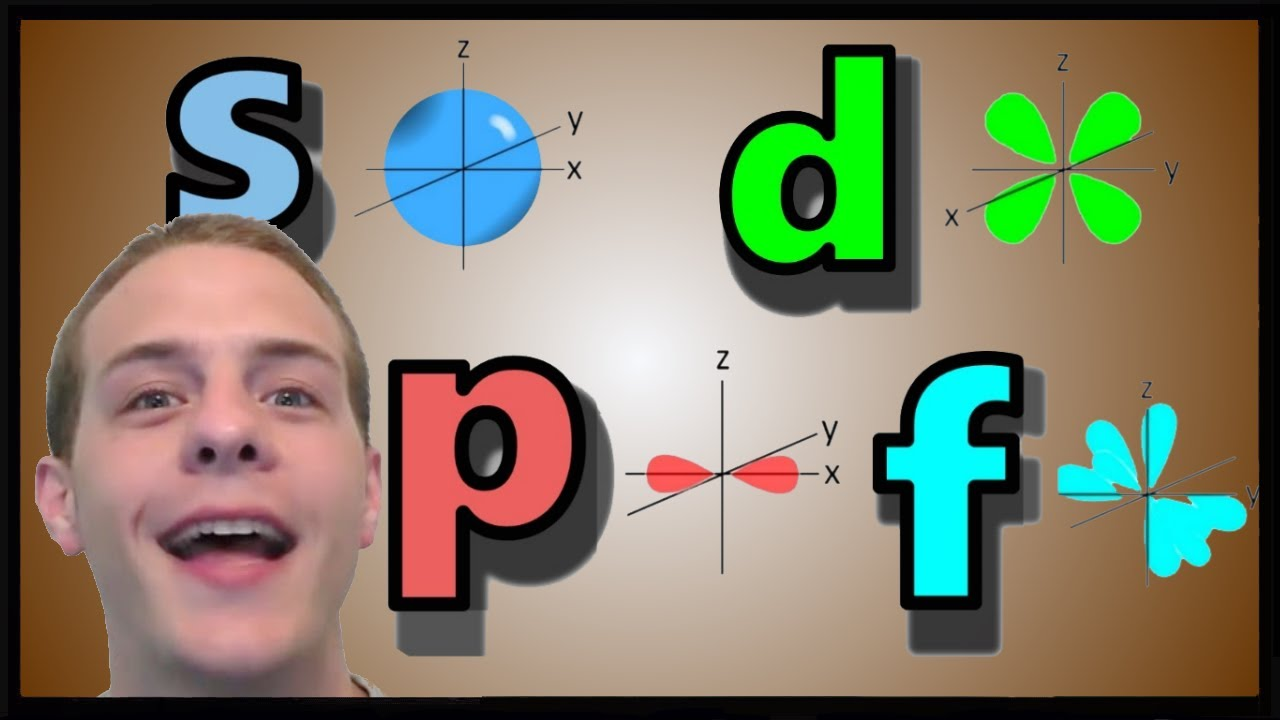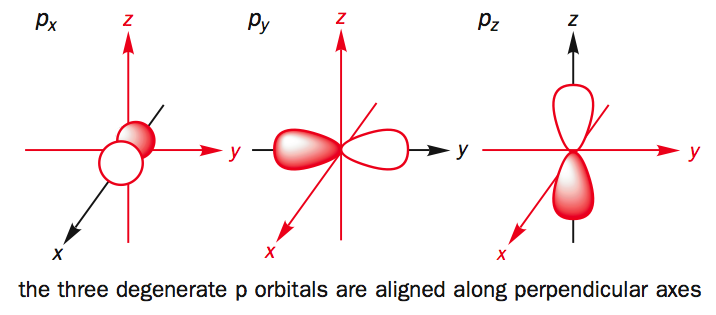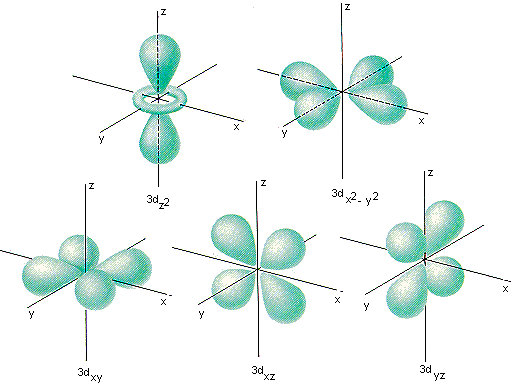orbitalOrbit

A circular path at a definite distance from the nucleus in which electron revolves around the nucleus is called orbit.

n=1,2,3,4,….., etc.

Orbital

The region in three dimensional space around the nucleus where the probability of finding electron is maximum, is called orbital.

## Shape of Atomic Orbitalssource : www.dirk bertel.com

## s-Orbital

It is spherical is shape, in which probability of finding electron is identical in all directions from the nucleus. It has only one orientation .  s-orbitals are spherically symmetrical so electron density is not concentrated along any axis. All s-orbitals are spherical (similar) shape but size increases with increase in value of Principal Quantum No.source : en.wiki books.com

## p-Orbitals :

The p- orbital is dumb-bell in shape, it consists of two lobes, one on each side of the nucleus. The probability of finding an electron is equal is both the lobes. The p-orbitals have been denoted as px , py & pz depending upon the orientation.source : liver pool.ac.uk

## d-orbitals

The five d-orbitals are equivalent in energy ,its shape is like double dumb-bell. The d-orbitals are denoted as dxy, dyz, dzx, dx2-y2 & dz2 , in presence of magnetic field d-orbitals split into two groups such as dxy, dyz, dzx, or t2g & dx2-y2 & dz2 or eg

source: chemed.chem.purdue.edu## f-orbitals-

The seven f-orbitals are equivalent in energy. The orbitals are denoted as

fz3 fxz2 fyz2 fxyz fz(x2−y2) fx(x2−3y2) fy(3x2−y2

Their shape is very complicated.en.wikibooks.org